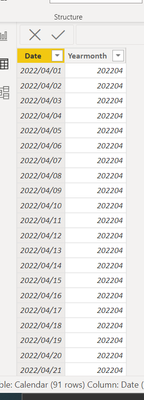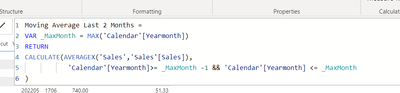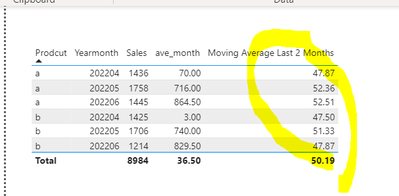cancel
Showing results for
Did you mean:Helper I

## How to get moving average on monthly

HI, all

I'd like to get monthly moving average by making measure as below, but it may not work well

------------------------------------------------------------

<Measure>

ave_month = CALCULATE(AVERAGEX(crossjoin(VALUES('Calendar'[Yearmonth]),VALUES('Sales'[Prodcut])),'mea'[sum]),DATESINPERIOD('Calendar'[Date],MIN('Calendar'[Date]),-1,MONTH))

<Model
><Table>
SalesCalendar------------------------------------------------------------

10 REPLIES 10Helper I

@mangaus1111

Sorry I was wrong

What I'd like to get is 12 (average of sum of April "10" and sum of May 14 )Helper I

@mangaus1111

Thanks a million!

Based on your sample, what I'd like to get is 5.5(average of April 5 and May 6)Solution Sage

then in the end what you want is the moving average of a moving averageHelper I

@mangaus1111But, result is not as I expected.It seems that average is calculated on a daily basis not monthly.Solution Sage

Is your model not like this?Solution Sage

Hi @taimaizumi ,

try this measure

``````Moving Average Last 2 Months =
VAR _MaxMonth = MAX('Date'[Year*Month])
RETURN
CALCULATE(AVERAGEX('Facts5','Facts5'[Sales]),
'Date'[Year*Month]>= _MaxMonth -1 && 'Date'[Year*Month] <= _MaxMonth
)``````

If this post helps, then please consider Accept it as the solution to help the other members find it more quickly.Helper I

HI, @mangaus1111

Sorry, my explanation was not enough
What I'd like to get is "moving" average among this month and previous month by month and productSolution Sage

But if the granularity of your report is Product and Month, then you need a simple Averagex.

Avg = AVERAGEX('Sales','Sales'[Sales])

If this post helps, then please consider Accept it as the solution to help the other members find it more quickly.Helper I

Hi @mangaus1111

Thank you for you quick response.

Output image is below.
I'd like to get result by monthly and products.Solution Sage

Hi @taimaizumi ,

in the row of the matrix do you want Day and Product or Month and Product?